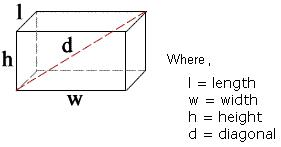## Rectangular Cuboid Calculator

 Length = units Width = units Height = units surface area = square units space diagonal = units volume = cubic units significant figures: 3 4 5 6 7 8 9

Rectangular Cuboid to find out the Total Surface Area and Volume for the given input values of Length, Width and Height.### Rectangular Cuboid Formulas

Rectangular Prism Volume = Length x Width x Height

Rectangular Prism Surface Area = 2(lw) + 2(hl) + 2(hw)

Rectangular Prism Diagonal Length = Root of (l2 + w2 + h2)

Rectangular Prism Perimeter = 2l + 2w

The geometry calculations of Rectangular Prism can be calculated in different units by multiplying respective value to the actual output. These are all the below values are used to convert the output in different units

Thinkcalculator.com provides you helpful and handy calculator resources.